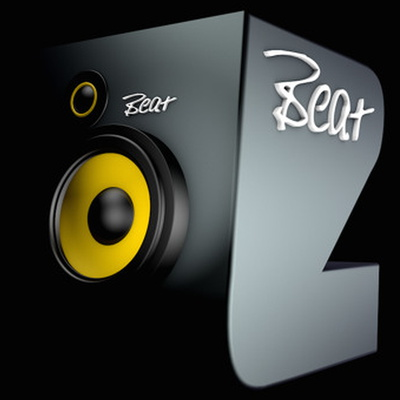-• Lyrics
• Information
• Top Tracks
• Related Tracks
• Related Artist

Tynan - UNDONE (with Aaron Gillespie)

[Pre-Chorus: Aaron Gillespie]
Let me out
Let me out
Let me out
Let me out
Let me out
Let me out
Let me out

[Chorus: Aaron Gillespie]
I'm lost inside this crazy broken place
(Let me out)
Being lost is what fuels my hate
(Let me out)
I'm dying to just to make you afraid
(Let me out)
[Build]
This is where
This is where
This is where I come (Undo—)

[Drop]
This is where
This is where
This is where I come undo—
This is wherе
This is where
This is wherе I come undone
Where I come undone

[Bridge: Aaron Gillespie]
Let me out
Let me out
Let me out

[Chorus: Aaron Gillespie]
I'm lost inside this crazy broken place
(Let me out)
Being lost is what fuels my hate
(Let me out)
I'm dying to just to make you afraid
(Let me out)
You might also like[Build]
This is where, this is where, this is where
This is where, this is where, this is where
This is where, this is where, this is where
This is where, this is where, this is where
This is where

[Pre-Drop]
Where I come undone

[Drop]
This is where
This is where
This is where I come undo—
This is where
This is where
This is where I come undone